Ch 4. The Mean Value Theorem Multimedia Engineering Math Maximum & Minimum Rolle'sTheorem Mean ValueTheorem MonotonicFunctions First DerivativeTest Concavity &Inflection SecondDerivative Test
 Chapter 1. Limits 2. Derivatives I 3. Derivatives II 4. Mean Value 5. Curve Sketching 6. Integrals 7. Inverse Functions 8. Integration Tech. 9. Integrate App. 10. Parametric Eqs. 11. Polar Coord. 12. Series Appendix Basic Math Units Search eBooks Dynamics Fluids Math Mechanics Statics Thermodynamics Author(s): Hengzhong Wen Chean Chin Ngo Meirong Huang Kurt Gramoll ©Kurt GramollMATHEMATICS - CASE STUDY SOLUTION Judy took part in a remote control motorcycle race and realized she needs to better understand its operation hso she can improve the motocycle's performance. She recorded some data so she cn construct a diagram of the displacement curve. The recorded data includes: The race totally lasts 30 seconds. The acceleration is negative between 10 to 22 seconds and is positive for the rest periods of time. The velocity changes from negative to positive at 14 seconds, and from positive to negative at 7 and 26 seconds. The velocity at 7, 14 and 22 are 0. Let the displacement curve starts from 0.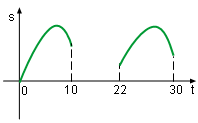Displacement Curve in (0, 10) and (22, 30) The second derivative test states: if the second derivative of a function is larger than 0, then the graph of the function is concave upward. It is given that the acceleration is positive between (0, 10) and between (22, 30), and the acceleration is the second derivative of the displacement with respect to time. In other words, the second derivative of displacement function is larger than 0. Therefore, the displacement curve is concave upward in these two interval as shown.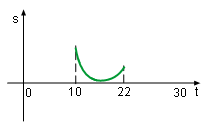Displacement Curve in (10, 22) The second derivative test also states: if the second derivative of a function is less than 0, then the graph of the function is concave downward. It is given that the acceleration is negative between (10, 22), and the acceleration is the second derivative of the displacement with respect to time. In other words, the second derivative of displacement function is smaller than 0. Therefore, the displacement curve is concave downward in this interval as shown in.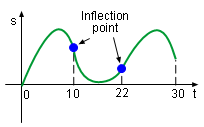Displacement Curve in (0, 30) Combining the above three curves, the displacement curve between 0 to 30 seconds is obtained. In this curve, there are two inflection points, t = 10 and t = 22 as shown.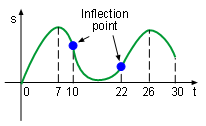Displacement Curve with Maximum Value Point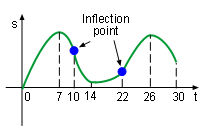Displacement Curve The velocity is the the first derivative of displacement with respect to time. When time is at 7 and 26 second, the velocity is 0. This satisfy the definition of critical points which requires the first derivative to be 0 or does not exist. In other word, 7 and 26 are the critical points of the displacement function. The first derivative test states: If the value of the function's first derivative changes from positive to negative at a critical point, then the function has a local maximum at this point. It is given that the velocity changes from positive to negative at 7 and 26 seconds which satisfies the first derivative test, and thus, the displacement of the function is maximum in these two points as shown in Displacement Curve with Maximum Value Point. Similarly, it can be found that 14 is the local minimum point as shown in Displacement Curve.